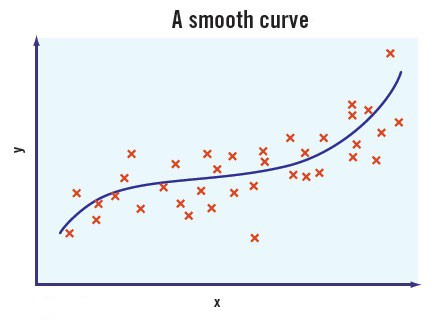# Linearization

When you trade financial markets you quickly learn that 10% up and 10% down are not the same thing!…

If we were to grow \$100 in 1000 incremental steps of 0.0095315% we will arrive at \$110.  And if we subsequently unwind/decline from this \$110 in 1000 incremental steps of 0.0095315% we will arrive back at \$100.  If however we were to grow the same \$100 to \$110 in one incremental step of 10%, and then decline from \$110 in one incremental step of 10% we will not arrive back at \$100 but at \$99 instead.

So why is this?  The reason is simple.  Because we are rounding out very tiny errors on each step, smooth incremental change will synchronize up and down movements; but because large errors are too big to be rounded out, coarse incremental change will not.

Mathematical Linearization

In the simplest of mathematical terms an increase of x% followed by a decrease of x% is the same as multiplying \$100 by the product of (1 + x) times (1 – x) which can be written mathematically as

\$100(1 + x)(1 – x)   =   \$100(1 – x2)   =   \$100 – \$100(x2)

Now if x is 10% then

\$100 – \$100(0.102)  =  \$100 – \$1.00  =  \$99.00

However if x is 0.0095315% then

\$100 – \$100(0.0000953152)  =  \$100 – \$0.00000009  =  \$100.00

So when x is small we can ignore the (x2) term, because this tiny error is negligible.

The process of linearization basically says that when x is small we can ignore the (x2) term, because this tiny error is negligible.  So when the error is small we are effectively resetting to “no error” on each step.

Cornerstone of Mathematical Science

Now believe it or not, “ignoring the (x2) term” is the cornerstone of all mathematical science. To ignore the (x2) term is simply to ignore “insignificant deviations” and this idea is referred to as  “linear approximation” or “linearization”.

The two primary tools of linearization are

1. Pure Continuity: Breaking all incremental change down into an “infinite number” of tiny infinitesimal steps (a simple example of which we have seen above).
2. The Law of Large Numbers: Dealing with an “infinitely large number” of things, so that we can confidently compress all information into an “average” (a modest example of which is the line-fitting graph shown below).Linear Approximation is used in virtually every area of science; from physics to engineering, from economics to ecology.  By eliminating insignificant deviations we can approximate the behaviour of a “nonlinear” system as being “linear” – which allows us to “compress” this behaviour into neat “solvable” mathematical equations.

Without this process of linearization the mathematical description of systems would be unsolvable, which effectively means that “without linearization nothing would be predictable”.  Thus we can say that

Linearization and Linear Dynamics have, to-date, been the cornerstone of all of mathematical science.